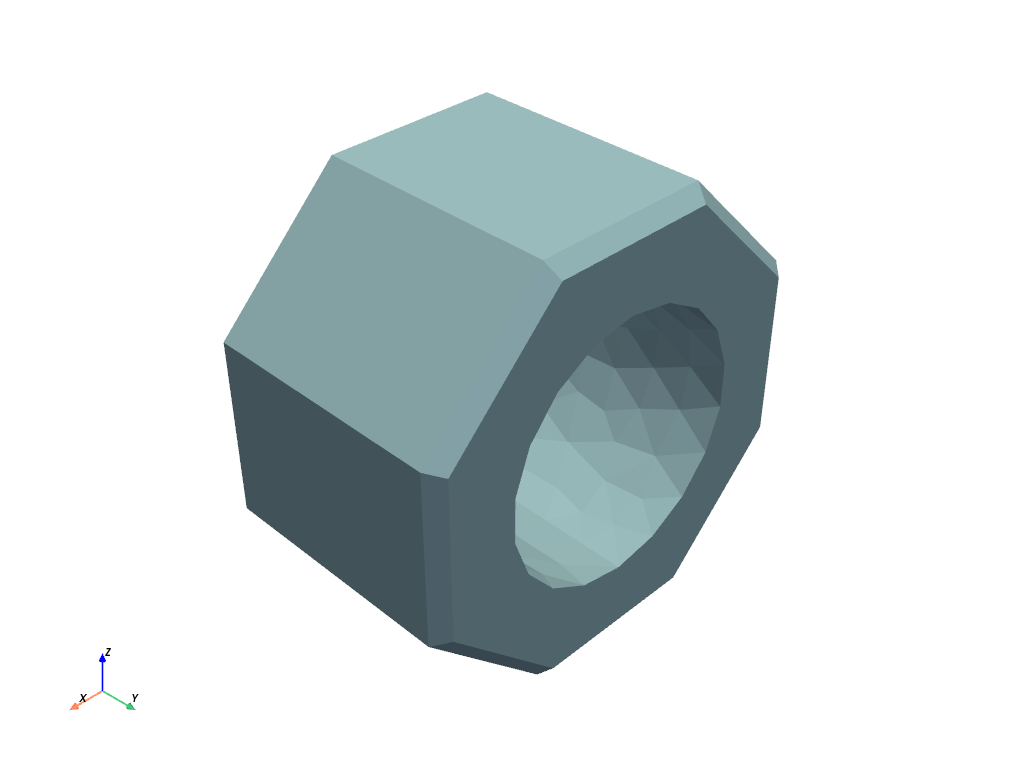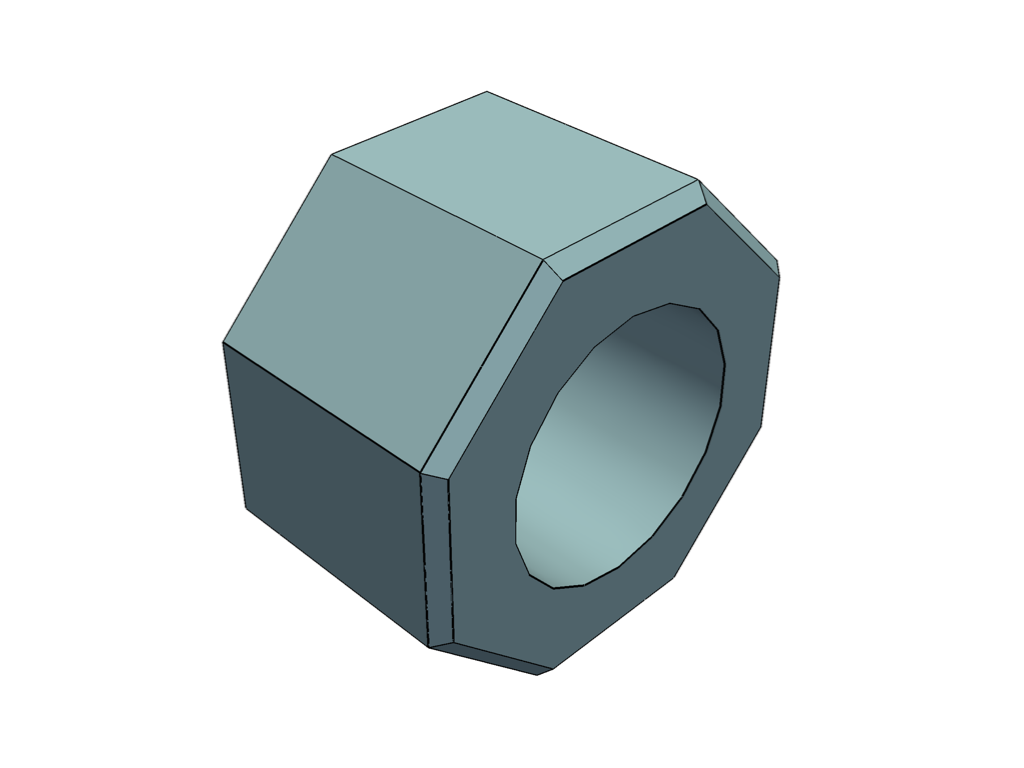```import pyvista
from pyvista import examples
```

This is a plot with the default flat shading.

```mesh = examples.load_nut()
mesh.plot()
```Here’s the same sphere with smooth shading.

```mesh.plot(smooth_shading=True)
```Note how smooth shading makes edges that should be sharp look odd, it’s because the points of these normals are averaged between two faces that have a sharp angle between them. You can avoid this by enabling `split_sharp_edges`.

Note

You can configure the splitting angle with the optional `feature_angle` keyword argument.

```mesh.plot(smooth_shading=True, split_sharp_edges=True)
```We can even plot the edges that will be split using `extract_feature_edges`.

```# extract the feature edges exceeding 30 degrees
edges = mesh.extract_feature_edges(
boundary_edges=False, non_manifold_edges=False, feature_angle=30, manifold_edges=False
)

# plot both the edges and the smoothed mesh
pl = pyvista.Plotter()
pl.enable_anti_aliasing()
pl.show()
```The `split_sharp_edges` keyword argument is compatible with physically based rendering as well.

```# plot both the edges and the smoothed mesh
pl = pyvista.Plotter()
pl.enable_anti_aliasing()
pl.add_mesh(mesh, color='w', split_sharp_edges=True, pbr=True, metallic=1.0, roughness=0.5)
pl.show()
```Total running time of the script: ( 0 minutes 1.660 seconds)

Gallery generated by Sphinx-Gallery